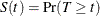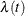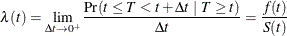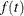Failure Time Distribution

Let T be a nonnegative random variable that represents the failure time of an individual from a homogeneous superpopulation. The survival distribution function (also known as the survivor function) of T is written asA mathematically equivalent way of specifying the distribution of T is through its hazard function. The hazard functionspecifies the instantaneous failure rate at t. If T is a continuous random variable,is expressed aswhereis the probability density function of T.